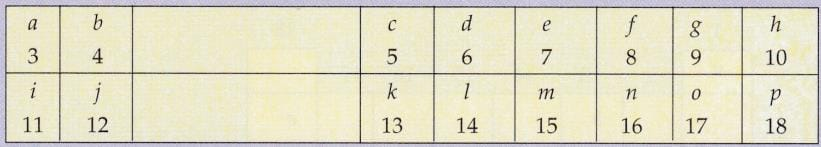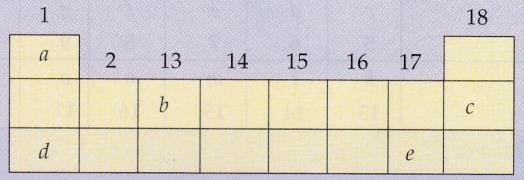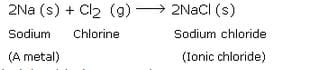Lakhmir Singh & Manjit Kaur: Periodic Classification Of Elements, Solutions- 4

# Lakhmir Singh & Manjit Kaur: Periodic Classification Of Elements, Solutions- 4 - Science Class 10

(Page No - 307)

Question 63:
The following diagram shows a part of the periodic table in which the elements are arranged according to their atomic numbers. (The letters given here are not the chemical symbols of the elements):(i) Which element has a bigger atom, a or ƒ ?
(ii)Which element has a higher valency, k or o?
(iii) Which element is more metallic, i or k?
(iv) Which element is more non-metallic, d or g?
(v) Select a letter which represents a metal of valency 2.
(vi) Select a letter which represents a non-metal of valency 2.
Solution :

(i) a (size decreases from left to right in a period) .
(ii) k (valency of k = 3; valency of o = 1).
(iii) i (metallic character decreases from left to right in a period).
(iv) g (non-metallic character increases from left to right in a period) .
(v) b (or j) .
(vi) f (or n) .

Question 64:
An element X is in group 2 of the periodic table :
(a) What will be the formula of its chloride ?
(b) What will be the formula of its oxide ?
Solution :

Valency of X = 2
(a) XCl 2
(b) XO

Question 65:
An element Y is in second period and group 16 of the periodic table :
(i) Is it a metal or non-metal ?
(ii) What is the number of valence electrons in its atom ?
(iii) What is its valency ?
(iv) What is the name of the element ?
(v) What will be the formula of the compound formed by Y with sodium ?
Solution :

(i) Non-metal .
(ii) 6 .
(iii) 2 .
(iv) Oxygen .
(v) Na 2 Y .

Question 66:
(a) An element X has mass number 40 and contains 21 neutrons in its atom. To which group of the periodic table does it belong ?
(b) The element X forms a compound X2Y. Suggest an element that Y might be and give reasons for your choice.
Solution :

(a) Group 1 (2, 8, 8, 1).
(b) Oxygen (X is monovalent so Y has to be divalent to form the compound X2Y)

Question 67:
An element X combines with oxygen to form an oxide XO. This oxide is electrically conducting.
(a) How many electrons would be there in the outermost shell of the element X ?
(b) To which group of the periodic table does the element X belong ?
(c) Write the formula of the compound formed when X reacts with chlorine.
Solution :

(a) 2.
(b) Group 2 .
(c) XCl 2 .

Question 68:
An element A has an atomic number of 6. Another element B has 17 electrons in its one neutral atom.
(a) n which groups of the periodic table would you expect to find these elements ?
(b) What type of bond is formed between A and B ?
(c) Suggest a formula of the compound formed between A and B.
Solution :

(a) A in group 14; B in group 17.
(b) Covalent bond.
(c) AB 4 .

Question 69:
The elements A, B, C and D belong to groups 1, 2,14 and 17 respectively of the periodic table. Which of the following pairs of elements would produce a covalent bond ?
(i) A and D (ii)C and D (iii) Aand B (iv)B and C (v) Aand C
Solution :

C and D .

Question 70:
An element X from group 2 reacts with element Y from group 16 of the periodic table.
(a) What is the formula of the compound formed ?
(b) What is the nature of bond in the compound formed ?
Solution :

(a) XY.
(b) Ionic bond .

Question 71:
A metal X is in the first group of the periodic table. What will be the formula of its oxide ?
Solution :

V alency of group 1 metals is 1 so it will react with oxygen (valency = 2) to form X2O.

Question 72:
An element A from group 14 of the periodic table combines with an element B from group 16.
(i) What type of chemical bond is formed ?
(ii) Give the formula of the compound formed.
Solution :

(i) Covalent bond is formed between two non-metals (A and B).
(ii) AB 2 .

Question 73:
An element X from group 2 of the periodic table reacts with an element Y from group 17 to form a compound.
(a) What is the nature of the compound formed ?
(b) State whether the compound formed will conduct electricity or not.
(c) Give the formula of the compound formed.
(d) What is the valency of element X ?
(e) How many electrons are there in the outermost shell of an atom of element Y ?
Solution :

(a) Ionic compound.
(b) Yes .
(c) XY 2 .
(d) 2 .
(e) 7 .

(Page No - 308)

Question 74:
The following diagram shows a part of the periodic table containing first three periods in which five elements have been represented by the letters a, b, c, d and e (which are not their chemical symbols):(i) Select the letter which represents an alkali metal.
(ii) Select the letter which represents a noble gas.
(iii) Select the letter which represents a halogen.
(iv) What type of bond is formed between a and e?
(v) What type of bond is formed between d and e ?
Solution :

(i) d.
(ii)c.
(iii) e.
(iv) Covalent bond .
(v) Ionic bond .

Question 75:
The elements A, B and C belong to groups 1, 14 and 17 respectively of the periodic table.
(a) Which two elements will form a covalent compound ?
(b) Which two elements will form an ionic compound ?
Solution :

(a) B and C.
(b) A and C .

Question 76:
Find the neutral atom in the periodic table which has the same number of electrons as K+ and Cl . What is this number ?
Solution :

Argon atom, 18 electrons .

Question 77:
Atoms of eight elements A, B, C, D, E, F, G and H have the same number of electron shells but different
number of electrons in their outermost shells. It was found that elements A and G combine to form an ionic compound. This ionic compound is added in a small amount to almost all vegetables and dishes during
cooking. Oxides of elements A and B are basic in nature while those of elements E and F are acidic. The
oxide of element D is, however, almost neutral. Based on the above information, answer the following questions :
(a) To which group or period of the periodic table do these elements belong ?
(b) What would be the nature of compound formed by a combination of elements B and F ?
(c) Which two of these elements could definitely be metals ?
(d) Which one of the eight elements is most likely to be found in gaseous state at room temperature ?
(e) If the number of electrons in the outermost shell of elements C and G be 3 and 7 respectively, write the formula of the compound formed by the combination of C and G.
Solution :

(a) 3rd period.
(b) Ionic compound .
(c) A and B.
(d) H.
(e) CG3 .

Question 78:
Write the names and symbols of two very reactive metals belonging to group 1 of the periodic table. Explain by drawing electronic structure, how either one of the two metals reacts with a halogen. With which name is the bond formed between these elements known and what is the class of the compound so formed known ? State any four physical properties of such compounds.
Solution :

Sodium (Na) and Potassium (K);
Sodium (Na) is a metal. So, sodium readily reacts with a halogen like chlorine (Cl) to form an ionic chloride called sodium chloride. This is illustrated below:Ionic bond; Ionic compounds .
Physical properties of ionic compounds:
(i) Ionic comp ounds are usually hard, brittle.
(ii) They conduct electricity when molten or dissolved.
(iii) They have high melting and boiling points.
(iv) Most are soluble in polar solvents such as water.

Question 79:
The non-metal A is an important constituent of our food and most of the fuels around us. A forms two oxides B and C. The oxide B is poisonous whereas oxide C causes global warming.
(a) Identify A, B and C.
(b) To which group of periodic table does A belong ?
(c) Name another element which is placed in the same group as A.
Solution :

(a) A is carbon (C); B is carbon monoxide (CO) ; C is carbon dioxide (CO 2 ).
(b) 14 th group .
(c) Silicon (Si) .

Question 80:
A non-metal X which is the largest constituent of air combines with hydrogen when heated in the presence of iron as catalyst to form a gas Y. When gas Y is treated with sulphuric acid, it forms a compound Z which is used as a chemical fertiliser.
(a) What are X, Y and Z ?
(b) To which group of periodic table does X belong ?
(c) Name the period of periodic table in which X is placed.
(d) Which element is placed just before X in the period ?
(e) Which element is placed just after X in the period ?
Solution :

(a) X is nitrogen gas, N2 ;
Y is ammonia gas, NH3
and Z is ammonium sulphate, (NH 4)2SO4.
(b) 15 th group .
(c) 2 nd period.
(d) Carbon, C .
(e) Oxygen, O.

The document Lakhmir Singh & Manjit Kaur: Periodic Classification Of Elements, Solutions- 4 | Science Class 10 is a part of the Class 10 Course Science Class 10.
All you need of Class 10 at this link: Class 10

## Science Class 10

78 videos|509 docs|153 tests

## FAQs on Lakhmir Singh & Manjit Kaur: Periodic Classification Of Elements, Solutions- 4 - Science Class 10

 1. What is periodic classification of elements?Ans. Periodic classification of elements refers to the arrangement of elements in the periodic table based on their atomic number, electronic configuration, and chemical properties. It allows for the systematic organization of elements, making it easier to study and understand their properties and trends.
 2. How are elements classified in the periodic table?Ans. Elements are classified in the periodic table based on their atomic number, which represents the number of protons in the nucleus of an atom. They are arranged in periods (rows) and groups (columns) based on their similar properties and electronic configuration.
 3. What are the advantages of periodic classification of elements?Ans. The advantages of periodic classification of elements include: 1. Easy organization: It provides a systematic way to organize and study the properties of elements, making it easier for scientists to understand and predict their behavior. 2. Identifying trends: The periodic table helps in recognizing patterns and trends in the properties of elements, such as atomic size, ionization energy, and electronegativity. 3. Predicting properties: By knowing the position of an element in the periodic table, we can predict its properties and behavior, which is useful in various fields, including chemistry, physics, and materials science.
 4. What is the significance of the periodic table in chemistry?Ans. The periodic table is of great significance in chemistry as it provides a wealth of information about the elements. It allows chemists to understand the relationships between different elements, their properties, and their behavior in chemical reactions. The periodic table also helps in predicting the reactivity, valency, and bonding patterns of elements, which are essential in studying and designing new compounds and materials.
 5. How does the periodic table help in understanding the periodicity of elements?Ans. The periodic table helps in understanding the periodicity of elements by arranging them in a way that shows the repetition of their properties at regular intervals. As we move across a period (row) in the periodic table, the properties of elements change in a systematic manner. Similarly, as we move down a group (column), elements with similar properties are found. This arrangement allows us to observe patterns and trends in properties such as atomic size, ionization energy, electron affinity, and chemical reactivity.

## Science Class 10

78 videos|509 docs|153 testsExplore Courses for Class 10 examSignup to see your scores go up within 7 days! Learn & Practice with 1000+ FREE Notes, Videos & Tests.
10M+ students study on EduRev
Track your progress, build streaks, highlight & save important lessons and more!
Related Searches

,

,

,

,

,

,

,

,

,

,

,

,

,

,

,

,

,

,

,

,

,

,

,

,

;